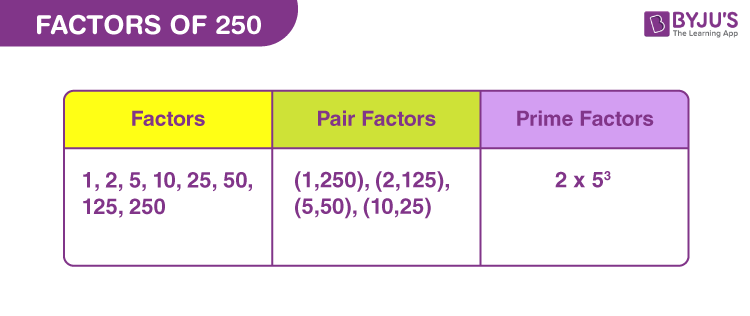# Factors of 250

In Mathematics, factors of 250 are the natural numbers by which we can evenly divide the original number. Since the factors divide the original number, therefore they are also called divisors of 250. 250 is a multiple of 25 but not a perfect square. Also, 250 is divisible by 5 (by divisibility rules).

If the multiplication of any two natural numbers is equal to 250, then they are the required factors of 250. 250 is an even composite number.

Let us find the factors of 250 along with pair factors and prime factors.## How to Find the Factors of 250?

It is simple to find the factors of 250. We need to divide 250 by all such natural numbers that can evenly divide the original number. Another method is to multiply two such numbers to get a result equal to 250.

 Division Method Multiplication Method 250 ÷ 1 = 250 1 × 250 = 250 250 ÷ 2 = 125 2 × 125 = 250 250 ÷ 5 = 50 5 × 50 = 250 250 ÷ 10 = 25 10 × 25 = 250 250 ÷ 25 = 10 25 × 10 = 250 250 ÷ 50 = 5 50 × 5 = 250 250 ÷ 225 = 2 225 × 5 = 250 250 ÷ 250 = 1 250 × 1 = 250

Therefore, the factors of 250 are 1, 2, 5, 10, 25, 50, 125, 250.

## Pair Factors of 250

When the product of two integers results in 250, then they are the required pair factors. Hence,

• 1 × 250 = 250
• 2 × 125 = 250
• 5 × 50 = 250
• 10 × 25 = 250

Therefore, the pair factors of 250 are (1, 250), (2, 125), (5, 50), (10, 25).

We can find the product of two negative numbers to get the factors in pairs, such that;

• -1 × -250 = 250
• -2 × -125 = 250
• -5 × -50 = 250
• -10 × -25 = 250

Therefore, the negative pair factors are (-1, -250), (-2, -125), (-5, -50), (-10, -25).

## Prime Factorisation of 250

Prime factorisation of 250 will result in the prime factors that can evenly divide the original number.

 Dividing 250 by smallest prime number 250 ÷ 2 = 125 Again dividing 125 by smallest prime number 125 ÷ 5 = 25 Dividing 25 by 5 25 ÷ 5 = 5 Again, dividing 5 by 5 5 ÷ 5 = 1

Therefore, the prime factors of 250 are 2 and 5.

 Prime factorisation of 250 = 2 x 5 x 5 x 5 Exponential form = 21 x 53

## Solved Examples

Q.1: What is the sum of all the factors of 250?

Solution: The factors of 250 are 1, 2, 5, 10, 25, 50, 125, 250.

Sum = 1+2+5+10+25+50+125+ 250 = 468

Therefore, the required sum is 468.

Q.2: What are the common factors of 255 and 250?

Solution: Let us list the factors of both the numbers 255 and 250.

250 → 1, 2, 5, 10, 25, 50, 125, 250

255 → 1, 3, 5, 15, 17, 51, 85, 255

Therefore, the common factors of 250 and 255 are 1 and 5.

Q.3: Find the GCF of 250 and 260.

Solution: The individual factors are:

250 → 1, 2, 5, 10, 25, 50, 125, 250

260 → 1, 2, 4, 5, 10, 13, 20, 26, 52, 65, 130, 260

Therefore, the greatest common factor of 250 and 260 is:

GCF (250, 260) = 10

### Practice Questions

1. Find the average of factors of 250.
2. Find the common factors of 250 and 150.
3. Is 20 a factor of 250?
4. Write the factors of 250 in product form.
5. Sheena wants to distribute 250 chocolates among 50 children in a classroom. How can she do that?

## Frequently Asked Questions on Factors of 250

### What are the factors of 250?

The factors of 250 are 1, 2, 5, 10, 25, 50, 125, 250.

### What are the prime factors of 250?

250 is divisible by only two prime factors, i.e. 2 and 5.

### Is 250 a composite number?

Yes, 250 is a composite number, because it has more than two factors.

### What are the multiples of 250?

Multiples of 250 are 250, 500, 750, 1000, 1250, 1500, 1750, 2000, 2250, 2500.

### What is the GCF of 150 and 250?

The respective factors are:
150 → 1, 2, 3, 5, 6, 10, 15, 25, 30, 50, 75, 150
250 → 1, 2, 5, 10, 25, 50, 125, 250
GCF (150, 250) = 50